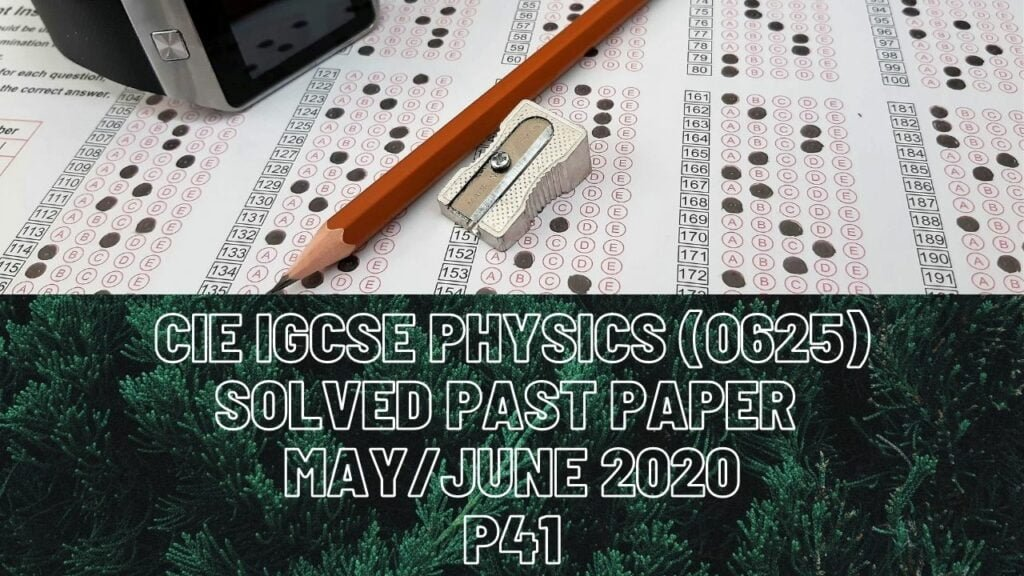IGCSE Physics### CIE IGCSE Physics Solved Past Paper May/June 2020 P41

2a) From 0 to 8 b) Force = constant*extension
Constant value = force/extension
= 8/15
= 0.53 N/cm
ci) Elastic potential energy
ii) Extension = force/constant
= (4/0.54)+(4/0.54)
= 14.8 =15 cm of extension
iii) So now extension is 6 instead of four here the increase in extension is
6-4=2. 2 is half of 4 so if new extension is half of the earlier extension,
New distance should also be half of the previous distance travelled
So distance = 15/2 = 7.5cm
3a) Same pressure is indicated by same level of liquid on both sides
b) Molecules move and collide with the walls of the container, due to
change of momentum force is exerted per unit area as
Pressure = force/area
ci) P1V1=P2V2
P2=P1V1/V2
(110^5)60/50 = 1.210^5 Pa of pressure (ii) density of liqui = ? Pressure of liquid = densitygraviy*height

Pressure of gas = atmospheric pressure+pressure of liquid
P2 = Patm + pgh
1.210^5 =110^5 + p15
Density = (1.210^5)-(110^5)/15
= 1.310^4 kgm/s 4a) fixed temperature at which liquid turns to gas

bi) Energy = specific heat capacity=mass*change in temperature = 42000.3*(100-95)
= 6300 J
ii) Thermal capacity = energy/change in temperature
= 6300/84
= 75 J/?
iii) Energy is required to work against intermolecular forces.
High energetic molecules escape from the surface of the liquid, leaving
behind less energetic molecules.
5a) Origin of rays that emerge parallel after passing through lense on
principal axis
bi) Enlarged,upright,virtual
6a) Audible range of frequency = 20Hz to 20KHz
So wavelength range = speed/frequency
= 340/20 +340/20000
= 17 to 0.017m
b) Longitudinal-vibrations are parallel to direction of wave motion and also
has rarefactions and compressions
Transverse-vibrations are perpendicular to direction of wave motion
c) Drum has low frequency which means longer wavelength so it travels
farther and diffracts more than the others
7 ai) It is a good conductor due to the mobile electrons in its structure
ii) Can be easily magnetised and demagnitised
bi) Alternating magnetic field produced in X due to alternating current emf is induced in Y by electromagnetic induction as coil in Y cuts magnetic field.
ii) Current in X is high to supply the power used in lamp
8a) Total emf = 9901.2 = 1188V number of single cells = total emf/emf of single cell = 1188/54 = 22 b(i) power = voltage*current
= 1.2**3.5
= 4.2 W
ii) Thick wires means less resistance which in turn decreases thermal
energy produces and so less heat is lost
9a) D-high and low voltage
A-variable voltage
bii) AND has output 1 only when both inputs are 1 OR produces output 1
always accept when inputs are both 0
ci) 0,0
ii) 2 inputs in NAND gate are 1, lower inout to C is 1, output is 0
10a) Number of protons
bii) they have less energy,mass and smaller charge.